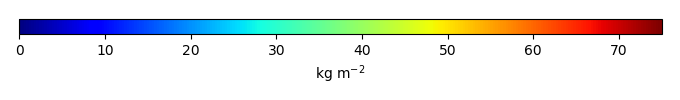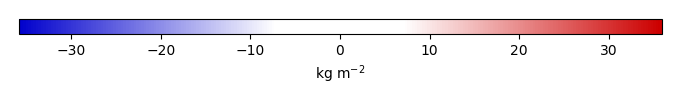# Mean State

Period Mean (original grids) [Pg]
Model Period Mean (intersection) [Pg]
Model Period Mean (complement) [Pg]
Benchmark Period Mean (intersection) [Pg]
Benchmark Period Mean (complement) [Pg]
Bias [kg m-2]
Bias Score 
Spatial Distribution Score 
Overall Score 
Benchmark [-] 34.1
CLM4 [-] 26.6 27.7 0.129 33.8 0.315 -1.13 0.70 0.95 0.82
CLM4.5 [-] 30.8 31.1 0.289 33.8 0.315 -0.144 0.67 0.91 0.79
CLM5 [-] 30.5 30.9 0.269 33.8 0.315 -0.226 0.73 0.95 0.84
Period Mean (original grids) [Pg]
Model Period Mean (intersection) [Pg]
Model Period Mean (complement) [Pg]
Benchmark Period Mean (intersection) [Pg]
Benchmark Period Mean (complement) [Pg]
Bias [kg m-2]
Bias Score 
Spatial Distribution Score 
Overall Score 
Benchmark [-] 44.8
CLM4 [-] 22.4 21.2 0.00988 44.4 0.478 -3.36 0.61 0.86 0.74
CLM4.5 [-] 16.6 15.8 0.0253 44.4 0.478 -4.14 0.53 0.69 0.61
CLM5 [-] 15.3 14.4 0.0241 44.4 0.478 -4.37 0.50 0.78 0.64
Period Mean (original grids) [Pg]
Model Period Mean (intersection) [Pg]
Model Period Mean (complement) [Pg]
Benchmark Period Mean (intersection) [Pg]
Benchmark Period Mean (complement) [Pg]
Bias [kg m-2]
Bias Score 
Spatial Distribution Score 
Overall Score 
Benchmark [-] 60.1
CLM4 [-] 25.6 25.1 0.326 59.3 0.762 -3.45 0.58 0.71 0.64
CLM4.5 [-] 30.0 29.1 0.783 59.3 0.762 -2.80 0.59 0.87 0.73
CLM5 [-] 28.7 27.8 0.749 59.3 0.762 -2.94 0.55 0.84 0.69
Period Mean (original grids) [Pg]
Model Period Mean (intersection) [Pg]
Model Period Mean (complement) [Pg]
Benchmark Period Mean (intersection) [Pg]
Benchmark Period Mean (complement) [Pg]
Bias [kg m-2]
Bias Score 
Spatial Distribution Score 
Overall Score 
Benchmark [-] 49.3
CLM4 [-] 7.10 7.08 0.133 48.3 0.997 -12.2 0.44 0.33 0.39
CLM4.5 [-] 66.4 65.5 1.03 48.3 0.997 6.85 0.48 0.26 0.37
CLM5 [-] 101. 99.2 1.16 48.3 0.997 17.5 0.43 0.12 0.28
Period Mean (original grids) [Pg]
Model Period Mean (intersection) [Pg]
Model Period Mean (complement) [Pg]
Benchmark Period Mean (intersection) [Pg]
Benchmark Period Mean (complement) [Pg]
Bias [kg m-2]
Bias Score 
Spatial Distribution Score 
Overall Score 
Benchmark [-] 55.7
CLM4 [-] 21.0 19.9 1.00 50.7 4.99 -6.63 0.65 0.16 0.40
CLM4.5 [-] 24.0 22.5 1.29 50.7 4.99 -4.84 0.67 0.37 0.52
CLM5 [-] 20.6 19.1 1.31 50.7 4.99 -6.12 0.65 0.27 0.46
Period Mean (original grids) [Pg]
Model Period Mean (intersection) [Pg]
Model Period Mean (complement) [Pg]
Benchmark Period Mean (intersection) [Pg]
Benchmark Period Mean (complement) [Pg]
Bias [kg m-2]
Bias Score 
Spatial Distribution Score 
Overall Score 
Benchmark [-] 131.
CLM4 [-] 62.4 60.7 0.139 130. 0.462 -7.63 0.58 0.50 0.54
CLM4.5 [-] 111. 108. 0.437 130. 0.462 -2.29 0.65 0.88 0.76
CLM5 [-] 133. 130. 0.561 130. 0.462 0.0856 0.64 0.88 0.76
Period Mean (original grids) [Pg]
Model Period Mean (intersection) [Pg]
Model Period Mean (complement) [Pg]
Benchmark Period Mean (intersection) [Pg]
Benchmark Period Mean (complement) [Pg]
Bias [kg m-2]
Bias Score 
Spatial Distribution Score 
Overall Score 
Benchmark [-] 153.
CLM4 [-] 67.8 68.9 0.471 151. 2.09 -4.24 0.57 0.87 0.72
CLM4.5 [-] 96.4 97.4 0.823 151. 2.09 -2.50 0.63 0.87 0.75
CLM5 [-] 91.8 93.6 0.579 151. 2.09 -2.83 0.62 0.90 0.76
Period Mean (original grids) [Pg]
Model Period Mean (intersection) [Pg]
Model Period Mean (complement) [Pg]
Benchmark Period Mean (intersection) [Pg]
Benchmark Period Mean (complement) [Pg]
Bias [kg m-2]
Bias Score 
Spatial Distribution Score 
Overall Score 
Benchmark [-] 58.4
CLM4 [-] 31.2 30.0 0.429 56.8 1.57 -3.14 0.65 0.93 0.79
CLM4.5 [-] 38.1 36.3 0.948 56.8 1.57 -1.83 0.67 0.82 0.74
CLM5 [-] 33.0 31.1 0.855 56.8 1.57 -2.67 0.66 0.86 0.76
Period Mean (original grids) [Pg]
Model Period Mean (intersection) [Pg]
Model Period Mean (complement) [Pg]
Benchmark Period Mean (intersection) [Pg]
Benchmark Period Mean (complement) [Pg]
Bias [kg m-2]
Bias Score 
Spatial Distribution Score 
Overall Score 
Benchmark [-] 59.4
CLM4 [-] 4.45 4.72 0.0498 58.9 0.523 -15.0 0.40 0.13 0.27
CLM4.5 [-] 66.3 66.8 0.532 58.9 0.523 2.79 0.52 0.47 0.50
CLM5 [-] 119. 120. 0.656 58.9 0.523 18.1 0.45 0.23 0.34
Period Mean (original grids) [Pg]
Model Period Mean (intersection) [Pg]
Model Period Mean (complement) [Pg]
Benchmark Period Mean (intersection) [Pg]
Benchmark Period Mean (complement) [Pg]
Bias [kg m-2]
Bias Score 
Spatial Distribution Score 
Overall Score 
Benchmark [-] 58.9
CLM4 [-] 17.9 17.1 0.623 57.4 1.53 -4.25 0.53 0.85 0.69
CLM4.5 [-] 25.7 24.2 1.35 57.4 1.53 -3.11 0.57 0.67 0.62
CLM5 [-] 27.6 25.8 1.60 57.4 1.53 -2.75 0.54 0.47 0.51
Period Mean (original grids) [Pg]
Model Period Mean (intersection) [Pg]
Model Period Mean (complement) [Pg]
Benchmark Period Mean (intersection) [Pg]
Benchmark Period Mean (complement) [Pg]
Bias [kg m-2]
Bias Score 
Spatial Distribution Score 
Overall Score 
Benchmark [-] 66.2
CLM4 [-] 19.0 18.5 0.338 64.6 1.58 -7.51 0.53 0.25 0.39
CLM4.5 [-] 47.1 45.7 0.803 64.6 1.58 -2.02 0.67 0.62 0.65
CLM5 [-] 44.2 42.7 0.830 64.6 1.58 -2.44 0.72 0.85 0.78
Period Mean (original grids) [Pg]
Model Period Mean (intersection) [Pg]
Model Period Mean (complement) [Pg]
Benchmark Period Mean (intersection) [Pg]
Benchmark Period Mean (complement) [Pg]
Bias [kg m-2]
Bias Score 
Spatial Distribution Score 
Overall Score 
Benchmark [-] 1.38e+03
CLM4 [-] 500. 493. 6.80 1.32e+03 56.4 -5.57 0.55 0.46 0.51
CLM4.5 [-] 984. 866. 117. 1.32e+03 56.4 -2.57 0.61 0.75 0.68
CLM5 [-] 1.06e+03 1.04e+03 16.5 1.32e+03 56.4 -1.32 0.60 0.43 0.51
Period Mean (original grids) [Pg]
Model Period Mean (intersection) [Pg]
Model Period Mean (complement) [Pg]
Benchmark Period Mean (intersection) [Pg]
Benchmark Period Mean (complement) [Pg]
Bias [kg m-2]
Bias Score 
Spatial Distribution Score 
Overall Score 
Benchmark [-] 64.3
CLM4 [-] 5.11 5.05 0.0898 63.6 0.726 -3.56 0.41 0.51 0.46
CLM4.5 [-] 9.44 9.22 0.283 63.6 0.726 -3.23 0.45 0.78 0.62
CLM5 [-] 14.0 13.8 0.386 63.6 0.726 -2.91 0.48 0.82 0.65
Period Mean (original grids) [Pg]
Model Period Mean (intersection) [Pg]
Model Period Mean (complement) [Pg]
Benchmark Period Mean (intersection) [Pg]
Benchmark Period Mean (complement) [Pg]
Bias [kg m-2]
Bias Score 
Spatial Distribution Score 
Overall Score 
Benchmark [-] 90.9
CLM4 [-] 15.0 14.8 0.156 89.5 1.44 -10.4 0.43 0.15 0.29
CLM4.5 [-] 69.6 68.4 0.838 89.5 1.44 -2.14 0.53 0.83 0.68
CLM5 [-] 123. 122. 1.25 89.5 1.44 5.76 0.44 0.42 0.43
Period Mean (original grids) [Pg]
Model Period Mean (intersection) [Pg]
Model Period Mean (complement) [Pg]
Benchmark Period Mean (intersection) [Pg]
Benchmark Period Mean (complement) [Pg]
Bias [kg m-2]
Bias Score 
Spatial Distribution Score 
Overall Score 
Benchmark [-] 42.5
CLM4 [-] 7.15 6.71 0.234 40.2 2.26 -9.74 0.46 0.21 0.34
CLM4.5 [-] 17.0 15.6 0.919 40.2 2.26 -5.66 0.57 0.70 0.64
CLM5 [-] 16.9 15.5 0.942 40.2 2.26 -5.56 0.57 0.73 0.65
Period Mean (original grids) [Pg]
Model Period Mean (intersection) [Pg]
Model Period Mean (complement) [Pg]
Benchmark Period Mean (intersection) [Pg]
Benchmark Period Mean (complement) [Pg]
Bias [kg m-2]
Bias Score 
Spatial Distribution Score 
Overall Score 
Benchmark [-] 45.4
CLM4 [-] 27.3 26.7 0.235 44.5 0.888 -3.94 0.69 0.37 0.53
CLM4.5 [-] 40.5 39.4 0.423 44.5 0.888 -0.617 0.68 0.87 0.77
CLM5 [-] 32.2 31.2 0.308 44.5 0.888 -2.77 0.72 0.77 0.75
Period Mean (original grids) [Pg]
Model Period Mean (intersection) [Pg]
Model Period Mean (complement) [Pg]
Benchmark Period Mean (intersection) [Pg]
Benchmark Period Mean (complement) [Pg]
Bias [kg m-2]
Bias Score 
Spatial Distribution Score 
Overall Score 
Benchmark [-] 88.1
CLM4 [-] 29.3 29.2 0.147 86.3 1.76 -10.5 0.53 0.29 0.41
CLM4.5 [-] 58.2 58.3 0.293 86.3 1.76 -4.85 0.64 0.58 0.61
CLM5 [-] 75.2 75.3 0.272 86.3 1.76 -1.69 0.63 0.87 0.75
Period Mean (original grids) [Pg]
Model Period Mean (intersection) [Pg]
Model Period Mean (complement) [Pg]
Benchmark Period Mean (intersection) [Pg]
Benchmark Period Mean (complement) [Pg]
Bias [kg m-2]
Bias Score 
Spatial Distribution Score 
Overall Score 
Benchmark [-] 34.9
CLM4 [-] 10.4 10.7 0.0171 34.7 0.216 -5.90 0.51 0.64 0.58
CLM4.5 [-] 13.2 13.8 0.0700 34.7 0.216 -5.01 0.57 0.87 0.72
CLM5 [-] 11.5 12.1 0.0886 34.7 0.216 -5.41 0.54 0.87 0.71
Period Mean (original grids) [Pg]
Model Period Mean (intersection) [Pg]
Model Period Mean (complement) [Pg]
Benchmark Period Mean (intersection) [Pg]
Benchmark Period Mean (complement) [Pg]
Bias [kg m-2]
Bias Score 
Spatial Distribution Score 
Overall Score 
Benchmark [-] 101.
CLM4 [-] 59.6 59.1 0.178 100. 0.811 -4.62 0.66 0.34 0.50
CLM4.5 [-] 69.1 68.6 0.387 100. 0.811 -3.21 0.70 0.58 0.64
CLM5 [-] 68.5 67.8 0.372 100. 0.811 -3.35 0.69 0.46 0.58
Period Mean (original grids) [Pg]
Model Period Mean (intersection) [Pg]
Model Period Mean (complement) [Pg]
Benchmark Period Mean (intersection) [Pg]
Benchmark Period Mean (complement) [Pg]
Bias [kg m-2]
Bias Score 
Spatial Distribution Score 
Overall Score 
Benchmark [-] 60.7
CLM4 [-] 31.0 31.1 0.187 60.4 0.300 -4.07 0.63 0.54 0.59
CLM4.5 [-] 36.9 36.7 0.416 60.4 0.300 -3.04 0.64 0.78 0.71
CLM5 [-] 47.2 47.5 0.479 60.4 0.300 -1.44 0.67 0.84 0.75
Period Mean (original grids) [Pg]
Model Period Mean (intersection) [Pg]
Model Period Mean (complement) [Pg]
Benchmark Period Mean (intersection) [Pg]
Benchmark Period Mean (complement) [Pg]
Bias [kg m-2]
Bias Score 
Spatial Distribution Score 
Overall Score 
Benchmark [-] 29.6
CLM4 [-] 7.81 7.58 0.114 28.2 1.46 -5.87 0.50 0.25 0.38
CLM4.5 [-] 11.6 11.2 0.196 28.2 1.46 -4.41 0.54 0.54 0.54
CLM5 [-] 15.7 14.8 0.272 28.2 1.46 -3.23 0.57 0.49 0.53

# Temporally integrated period mean

BENCHMARK MEANMODEL MEANBIASBIAS SCORESPATIAL TAYLOR DIAGRAMMODEL COLORS# Spatially integrated regional mean

MODEL COLORS# All Models

BenchmarkCLM4CLM4.5CLM5# Data Information

Approach: I just read the data from the original data file and flip the latitude arrange from original 90N->90S to 90S->90N.

Temporal resolution: yearly

General information: This product was derived from Harmonized World Soil Database (HWSD) v1.2 dataset.

Spatial resolution: 0.5x0.5 degree